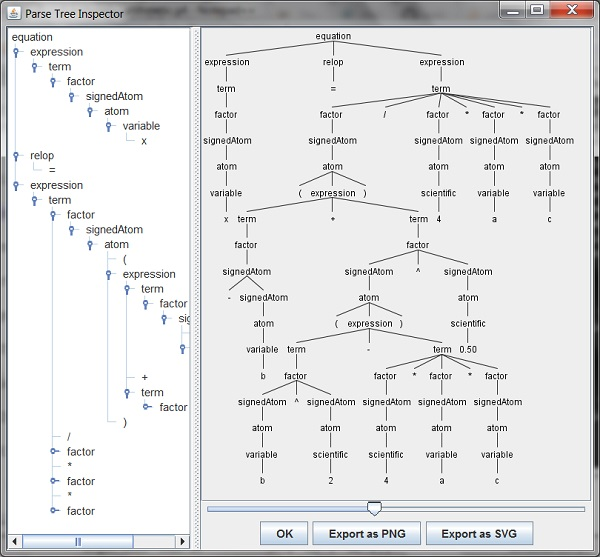ANTLR "Arithmetic" Grammar - Graphical Output

Q

Where to get a graphical output example of the "Arithmetic" grammar with ANTLR?

✍: FYIcenter

AYou can run the following ANTLR TestRig command to generate a graphical output from the "Arithmetic" lexer and parser:

```\fyicenter>java -cp .;antlr-4.7.1-complete.jar \
org.antlr.v4.gui.TestRig arithmetic equation -gui

x  = (-b + (b^2 -4*a*c)^0.50) / 4*a*c
^Z
```

The above test session will result the following graphical window alled "Parse Tree Inspector", displaying the parser output:Â

2021-01-09, 6603👍, 0💬